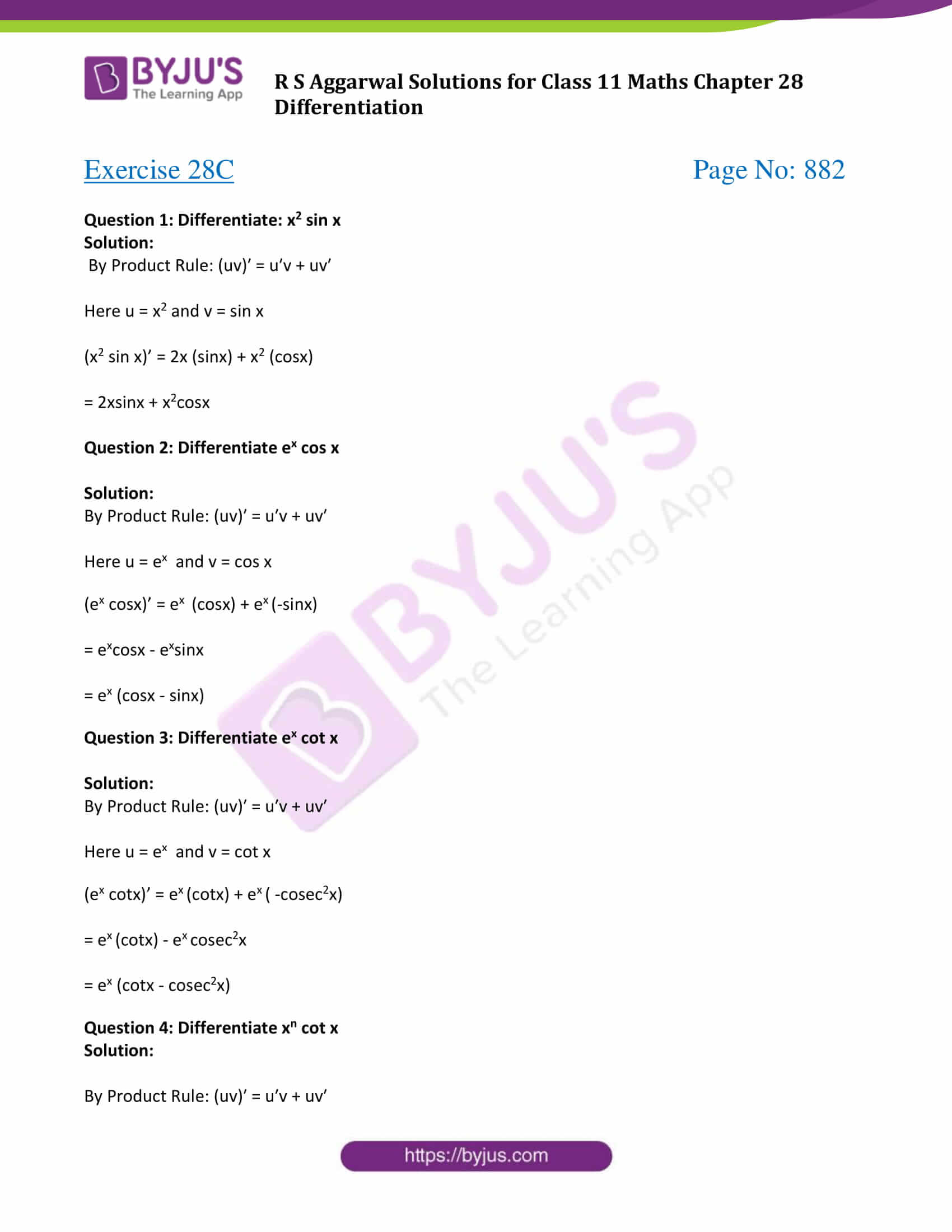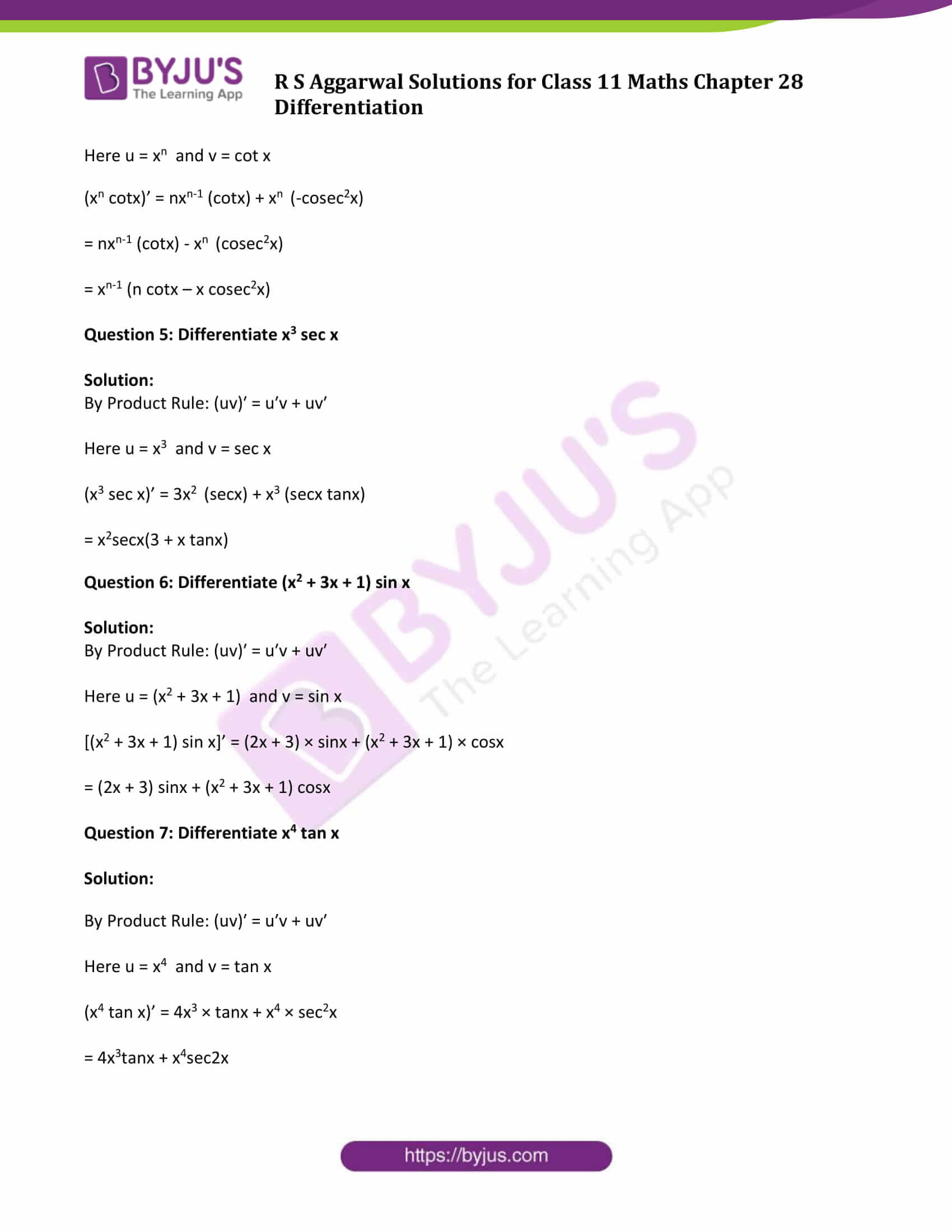# R S Aggarwal Solutions for Class 11 Maths Chapter 28 Differentiation Exercise 28C

Exercise 28C of Chapter 28 is about derivative of the product of functions, which states that, if f(x) and g(x) are two differentiable functions then f(x)g(x) is also differentiable.

By Product Rule: If u and v are any two functions then, (uv)′ = u′v + uv′

Class 11 Maths Chapter 28 Differentiation Exercise 28C is based on the topic: Derivative of the product of functions. Students are advised to download R S Aggarwal Class 11 Solutions and learn more about Differentiation in detail with the help of proven results.

## Download PDF of R S Aggarwal Solutions for Class 11 Maths Chapter 28 Differentiation Exercise 28C### Access Answers to Maths R S Aggarwal Class 11 Chapter 28 Differentiation Exercise 28C Page number 882

Question 1: Differentiate: x2 sin x

Solution:

By Product Rule: (uv)′ = u′v + uv′

Here u = x2 and v = sin x

(x2 sin x)’ = 2x (sinx) + x2 (cosx)

= 2xsinx + x2cosx

Question 2: Differentiate ex cos x

Solution:

By Product Rule: (uv)′ = u′v + uv′

Here u = ex and v = cos x

(ex cosx)’ = ex (cosx) + ex (-sinx)

= excosx – exsinx

= ex (cosx – sinx)

Question 3: Differentiate ex cot x

Solution:

By Product Rule: (uv)′ = u′v + uv′

Here u = ex and v = cot x

(ex cotx)’ = ex (cotx) + ex ( -cosec2x)

= ex (cotx) – ex cosec2x

= ex (cotx – cosec2x)

Question 4: Differentiate xn cot x

Solution:

By Product Rule: (uv)′ = u′v + uv′

Here u = xn and v = cot x

(xn cotx)’ = nxn-1 (cotx) + xn (-cosec2x)

= nxn-1 (cotx) – xn (cosec2x)

= xn-1 (n cotx – x cosec2x)

Question 5: Differentiate x3 sec x

Solution:

By Product Rule: (uv)′ = u′v + uv′

Here u = x3 and v = sec x

(x3 sec x)’ = 3x2 (secx) + x3 (secx tanx)

= x2secx(3 + x tanx)

Question 6: Differentiate (x2 + 3x + 1) sin x

Solution:

By Product Rule: (uv)′ = u′v + uv′

Here u = (x2 + 3x + 1) and v = sin x

[(x2 + 3x + 1) sin x]’ = (2x + 3) × sinx + (x2 + 3x + 1) × cosx

= (2x + 3) sinx + (x2 + 3x + 1) cosx

Question 7: Differentiate x4 tan x

Solution:

By Product Rule: (uv)′ = u′v + uv′

Here u = x4 and v = tan x

(x4 tan x)’ = 4x3 × tanx + x4 × sec2x

= 4x3tanx + x4sec2x

## Access other exercise solutions of Class 11 Maths Chapter 28 Differentiation

Exercise 28A Solutions

Exercise 28B Solutions

Exercise 28D Solutions

Exercise 28E Solutions